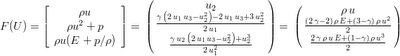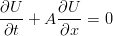## Saturday, January 24, 2009

### Convective Flux Jacobians

The Euler equations are an important system of equations in computational fluid dynamics (CFD). They are basically the full continuum equations of motion without the viscosity. Adding in the viscosity gives you the more realistic Navier-Stokes equations.The conserved variable vector isAnd the flux vector isThe flux Jacobian is simply the partial derivative of the flux with respect to the conserved variables (mass, momentum, energy).The maxima to calculate the Jacobian is straightforward:

U : matrix([u1], [u2], [u3]);

f1 : u2;
f2 : u2^2 / u1 + p_exp;
f3 : (u2 / u1) * (u3 + p_exp);
F : matrix([f1], [f2], [f3]);

A : zeromatrix(nd, nd);

for j : 1 thru nd do
(
for i : 1 thru nd do
(
A[i,j] : diff(F[i,1], U[j,1])
)
);

### Diagonalization

Using the chain rule lets you write the Euler equations in a quasi-linear form in terms of the flux Jacobian, now called A.The flux Jacobian can be diagonalized into a matrix of right eigenvectors, diagonal matrix of eigenvalues, and a matrix of left eigenvectors.The code to accomplish this in maxima is a little more involved, because of some substitutions needed to get everything in terms of u,gamma,a.

VLV : eigenvectors(simp_A);

a_exp : (g * (g - 1) / u1) * (u3 - u2^2 / (2 * u1));
a_exp : sqrt(a_exp);
simp_a : ratsimp(ev(a_exp, u1 = rho, u2 = rho * u, u3 = rho * E));
a_eqn : a^2 = simp_a^2;
c_exp : g*(2*E-u^2);
a_eqn : facsum(a_eqn, c_exp);

/* put the eigenvectors into a matrix: */
V : columnvector( VLV );
V : ratsimp( V );

/* put the eigenvalues into a diagonal matrix: */
L : diag( VLV );

/* substitute in the speed of sound: */
V : ratsimp(ratsubst(a, simp_a, ratsimp(V)));
V : factor(ratsimp(ratsubst(a, simp_a, rootscontract(V))));
/* factor out gammas */
V : facsum(V,g);
c_a : part(solve(ratsubst(c,c_exp,a_eqn),c),2);
V : ratsubst(c,c_exp,V);
V : ratsubst(ev(c_a),c,V);
V : facsum(V,u);

/* substitute in the speed of sound: */
L : ratsimp(ratsubst(a, simp_a, ratsimp(L)));

/* left eigenvectors: */
Vinv : factor( ratsimp( invert( V ) ) );
/* factor out gammas */
Vinv : facsum(Vinv,g);
d_exp : (g-1)/a^2;
Vinv : ratsubst(d,d_exp,Vinv);

### Conclusion

The thing to note about this result is that there are three distinct wave speeds in the one dimensional Euler equations, u-a, u+a, and u, where a is the speed of sound. There are lots of possible ways to write the eigenvectors, in terms of the enthalpy, or the energy, or the pressure, but the form above with only the flow velocity, the speed of sound, and the ratio of specific heats is pretty elegant. It really illustrates what a fundamental quantity the speed of sound is for this set of equations.

Chapter 3 of Riemann Solvers and Numerical Methods for Fluid Dynamics: A Practical Introduction has a good work-up of this development and shows some alternative formulations for the flux Jacobian.